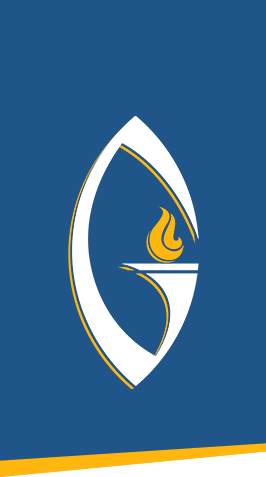## MATH 213: Math Concepts and Applications

MATH 213: Math Concepts and Applications Covers a variety of topics in math which may vary but generally are chosen from areas such as problem solving, algebra review, elementary statistics and probability, consumer math, voting methods, finance, number sense,...

## MATH 122: Statistics

MATH 122: Statistics Measures of central tendency and variability, probability, interval estimation, hypothesis testing, correlation and regression, and introduction to nonparametric techniques. 3 credits

## MATH 101: College Algebra

MATH 101: College Algebra Basic concepts of algebra, including real numbers, linear equations, polynomials, factoring, rational algebraic expressions, irrational equations, exponents and radicals, logarithmic and exponential functions, graphs of equations, quadratic...